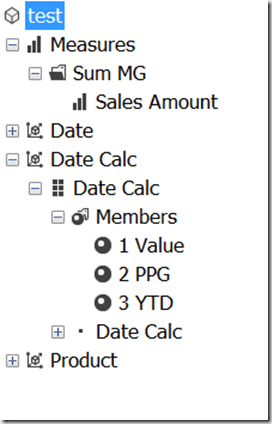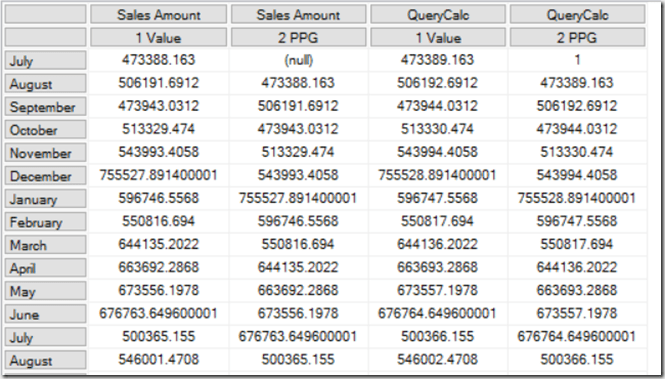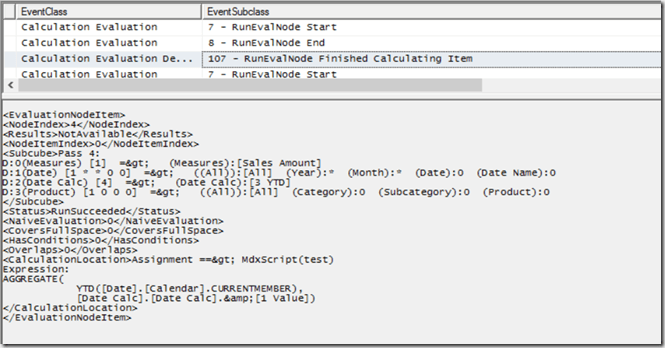# Finding Out Which MDX Calculations Are Being Evaluated By Your Query In Analysis Services Multidimensional, Part 2

In part 1 of this series I showed how you can use Profiler to find out which MDX calculations are being evaluated when a query runs on SSAS Multidimensional. In this post I’ll show a practical example of why this is so useful: a situation where SSAS evaluates a calculation that isn’t needed by a query.

Do you have a Date Tool dimension (also known as a Shell dimension or Time Utility dimension) in your cube? A lot of enterprise-level SSAS cubes use this technique to allow you to write a calculation once and have it apply to multiple measures. There are two main approaches to implementing Date Tool dimensions:

• You can create a dimension with one hierarchy and one real member and then use calculated members for your calculations, or
• You can create a dimension with one hierarchy and as many real members as you need calculations, and then use SCOPE statements on these members for your calculations

The second approach, described in detail in this article, is very popular but over the years I have seen several cases where customers of mine who use it have suffered from unexplained query performance problems, problems that have been solved by using the calculated member approach instead. It turns out that the Calculation Evaluation and Calculation Evaluation Detailed Information Profiler events can shed some light on the causes of these problems.

Here’s a simple test cube with a Date Tool dimension that has three real members on it:Here’s the contents of the MDX Script, copied from the Calculations tab in the Cube Editor in SSDT:

```CALCULATE;

SCOPE([Date Calc].[Date Calc].&[2 PPG]);
THIS = ([Date Calc].[Date Calc].&[1 Value],
[Date].[Calendar].CURRENTMEMBER.PREVMEMBER);
END SCOPE;

SCOPE([Date Calc].[Date Calc].&[3 YTD]);
THIS = AGGREGATE(
YTD([Date].[Calendar].CURRENTMEMBER),
[Date Calc].[Date Calc].&[1 Value]);
END SCOPE;
```

As you can see, two of the members on the [Date Calc] dimension are overwritten by scoped assignments: [2 PPG] is overwritten with a previous period growth calculation and [3 YTD] is overwritten by a year-to-date calculation.

Here’s a query that includes a calculated measure defined in the WITH clause and returns two out of three of the members on the [Date Calc] dimension – but does not return the [3 YTD] calculation:

```WITH
MEMBER [Measures].QueryCalc AS
[Measures].[Sales Amount] + 1

SELECT
{
[Measures].[Sales Amount],
[Measures].QueryCalc
}
*
{
[Date Calc].[Date Calc].&[1 Value],
[Date Calc].[Date Calc].&[2 PPG]
}
ON 0,
[Date].[Calendar].[Month].MEMBERS
ON 1
FROM
TEST
```Running a Profiler trace as described in my previous post reveals that when this query is run, not only are the [Query Calc] and [2 PPG] calculations evaluated, but [3 YTD] is evaluated too:It’s worth pointing out that this query was constructed deliberately to show a scenario where SSAS does decide to evaluate the [3 YTD] calculation, but in other cases it may decide otherwise. The reason it decides to do so here is due to a number of factors, including prefetching – see Jeffrey’s blog post here and the section on “Unexpected partition scans” here for some background information on this topic. Remember that in most cases prefetching is a good thing and is beneficial for performance, so if you see something like this happening in your cube you need to be sure that it’s actually causing you a performance problem before you try to prevent it.

If this is a problem for you there are a few things you can do. Rewriting your query to use subselects (if you have control over the MDX query that is being used) is one option:

```WITH
MEMBER [Measures].QueryCalc AS
[Measures].[Sales Amount] + 1

SELECT
{
[Measures].[Sales Amount],
[Measures].QueryCalc
}
*
{
[Date Calc].[Date Calc].MEMBERS
}
ON 0,
[Date].[Calendar].[Month].MEMBERS
ON 1
FROM
(SELECT
{
[Date Calc].[Date Calc].&[1 Value],
[Date Calc].[Date Calc].&[2 PPG]
}
ON 0
FROM
TEST)
CELL PROPERTIES VALUE
```

Using the following connection string properties also works, because it turns off prefetching:

```disable prefetch facts=true; cache ratio=1
```

…but as I said, this might hurt query performance in other ways.

Finally, as I said, using calculated members on your Date Tool dimension instead of the real members/scope statements approach will also work too. In my opinion this is the best solution since the problems with calculated member selection in Excel that caused problems for the calculated member Date Tool approach in the past were fixed a long time ago, and it will work even if you can’t change how your MDX queries are generated.

### 12 responses

1.mpr0st says:

Hello Chris.

Thank you for your blog, it’s full of knowledge for the sadly soon to be extinct art of multidimensional models and it’s saved my life countless times.

One question, is there any book/blog post which describes in detail the calculated member approach to Date Tool dimensions? I was hoping that your book Expert Cube Development with SSAS Multidimensional Models (2nd edition) could be such, but it appears that that book doesn’t cover that approach in detail.

Thank you for your valuable time.

Cheers.

•Chris Webb says:

Hmm, I thought we did talk about the calculated member approach… but if you need more detail, this is *mostly* good: http://www.obs3.com/pdf/A%20Different%20Approach%20to%20Time%20Calculations%20in%20SSAS.pdf

However the recommendation to use a referenced relationship is not good. You just need to add the dimension to the cube and have no relationship between it and any measure group.

•mpr0st says:

Thank you Chris, great resource.

2.Ibrahim says:

Is there an article or blog post about implementing the Date Tool dimension in SSAS tabular?

3.ihafidh says:

Is there an article on implementing the Date Tool dimension in SSAS tabular?

•Chris Webb says:

No, because it isn’t possible! There are similar techniques using IF() and SWITCH() but they miss the point – you can’t apply one calculation across multiple measures in DAX yet.

4.Cray says:

Hi Chris:

I’m new to MDX so the question might be silly but I’ml struggling to understand what does following statement really mean – “using calculated members on your Date Tool dimension instead of the real members/scope statements approach will also work too” ?

Does it mean “A Different Approach to Time Calculations in SSAS” approach as per published on SQLBI website could potentially cause performance issue and the solution would be adopting “ssas time intelligence wizard” approach?

Thanks
Cray

•Chris Webb says:

I don’t ever recommend using the “SSAS Time Intelligence” wizard, because it creates some really bad, inefficient MDX.

What the SQLBI Date Tool approach does is create a dimension with real members (coming from rows in a dimension table) for each of the calculations you want, and then using SCOPE statements to assign calculations to each of these real members. That is what I’m saying can be slow. The alternative is to create a dimension and hierarchy with just one real member, and then creating calculated members on the hierarchy for each of the calculations you need. Although this has some disadvantages compared to the SQLBI Date Tool approach, it avoids the performance problems I describe in this post.

5.Cray says:

Hi Chris:

•Chris Webb says: